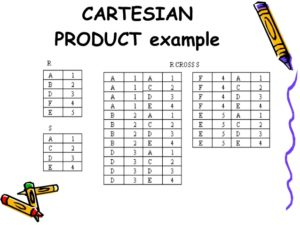# Relational Algebra

Relational Algebra

Algebra is a formal structure consisting of sets and operations on those sets. Relational algebra is a formal system for manipulating relations.

• Operands of this Algebra are relations.
• Operations of this Algebra include the usual set operations and special operations defined for relations such as :
• selection
• projection
• join

Set Operations on Relations

For the set operations on relations, both operands must have the same schema, and the result has that same schema.

1. R1 U R2 (union) is the relation containing all tuples that appear in R1, R2, or both.
2. R1 n R2 (intersection) is the relation containing all tuples that appear in both R1 and R2.
3. R1 – R2 (set difference) is the relation containing all tuples of R1 that do not appear in R2.Selection
Selects tuples from a relation whose attributes meet the selection criteria, which is normally expressed as a predicate.

R2 = select(R1,P)

That is, from R1 we create a new relation R2 containing those tuples from R1 that satisfy the predicate P.

A predicate is a Boolean expression whose operators are and, or, not and arithmetic comparisons (LT, LE, GT, GE, EQ, NE), and whose operands are either domain names or domain constants.

select(students,Class=XII)

Projection

Chooses a subset of the columns in a relation, and discards the rest.

R2 = project(R1,D1,D2,…Dn)

That is, from the tuples in R1 we create a new relation R2 containing only the domains D1,D2,..Dn.

Union
R UNION S

Includes all tuples that are in either R or S. Duplicate tuples are removed.

For a union operation r U s to be valid, two conditions must hold:

The relation r and s must be of the same arity, i.e. they must have the same number of attributes.

The domains of the ith attribute of r and the ith attribute of s must be the same for all i.

Example:

Join
Combines attributes of two relations into one.

R3 = join(R1,D1,R2,D2)

Given a domain from each relation, join considers all possible pairs of tuples from the two relations, and if their values for the chosen domains are equal, it adds a tuple to the result containing all the attributes of both tuples (discarding the duplicate domain D2).

Natural join: If the two relations being joined have exactly one attribute (domain) name in common, then we assume that the single attribute in common is the one being compared to see if a new tuple will be inserted in the result.

OUTER JOIN

Notice that much of the data is lost when applying a join to two relations. In some cases this lost data might hold useful information. An outer join retains the information that would have been lost from the tables, replacing missing data with nulls. There are three forms of the outer join, depending on which data is to be kept.

LEFT OUTER JOIN – keep data from the left-hand table
RIGHT OUTER JOIN – keep data from the right-hand table
FULL OUTER JOIN – keep data from both tables

Cartesian Product

The Cartesian Product is also an operator which works on two sets. It is sometimes called the CROSS PRODUCT or CROSS JOIN. It combines the tuples of one relation with all the tuples of the other relation.

ExampleSet Operations on Relations

For the set operations on relations, both operands must have the same schema, and the result has that same schema.

• R1 U R2 (union) is the relation containing all tuples that appear in R1, R2, or both.
• R1 n R2 (intersection) is the relation containing all tuples that appear in both R1 and R2.
• R1 – R2 (set difference) is the relation containing all tuples of R1 that do not appear in R2.